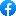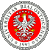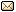XXXIX Workshop on Geometric Methods in Physics 19.06–25.06.2022 XI School on Geometry and Physics 27.06–1.07.2022
 Home First Announcement Second Announcement Travel and local information Participants of Workshop Participants of School Registration Photos Proceedings Previous Workshops EmailIn order to secure the right conditions for our Workshop we have decided to exceptionally move (only for this year's meeting) the site of our Workshop to the campus of our University in Białystok.

# Milan Niestijl

## Positive Energy Representations of Gauge Groups With Support at a Fixed Point.

Let $\mathcal{K} \to M$ be a locally trivial smooth bundle of Lie groups equipped with an action of some Lie group $P$ by bundle automorphisms. Complementing recent progress B. Janssens and K.H. Neeb on the case where the $P$-action on $M$ has no fixed-points, projective unitary representations $\overline{\rho}$ of the locally-convex Lie group $\mathcal{G} := \Gamma_c(\mathcal{K})$ are studied which are of "positive energy" and factor entirely through the germs at some fixed point $a \in M$ of the $P$-action. Under suitable assumptions, it is shown that the kernel of a particular quadratic form on $\mathbb R[[x_1,\cdots, x_d]]$ generates an ideal in $\mathfrak{G} := \mathcal{Lie}(\mathcal{G})$ on which the derived representation $d\rho$ must vanish. This leads in particular to sufficient conditions for $d\rho$ to factor through a finite jet space $J^k_x(\mathcal{K})$ or through $J^\infty_a(N, \mathcal{K})$ for some usually lower-dimensional submanifold $N$. Some examples are considered. If time permits, we will have a closer look at the special case where $P=S^1$.

 Event sponsored by: University of BialystokWebpage by: Tomasz Golinski